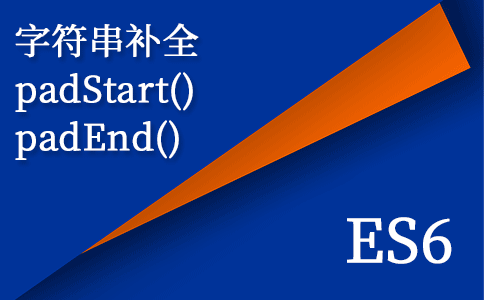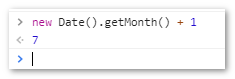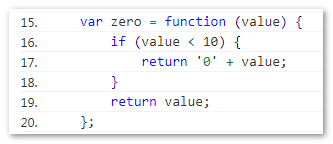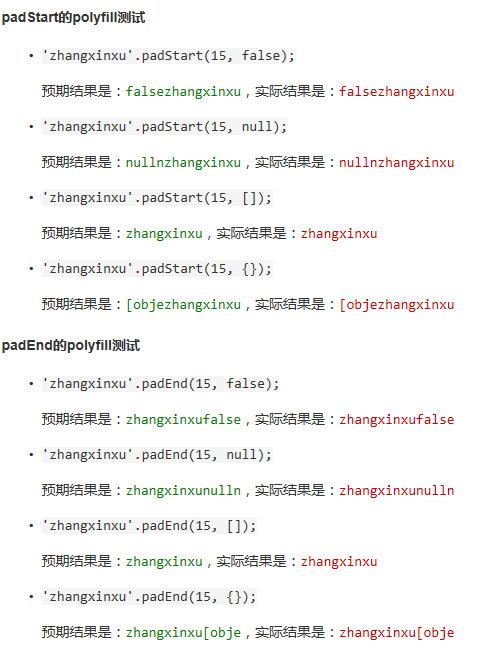β

# JS字符串补全方法padStart()和padEnd()简介

by zhangxinxu from https://www.zhangxinxu.com/wordpress/?p=7826

### 一、关于字符串补全``-07-23``

``var month = new Date().getMonth() + 1;    // 结果是7````````if (month < 10) {
month = '0' + month;
}``````### 二、关于padStart

``` padStart ``` 可以在字符串的开头进行字符补全。

``str.padStart(targetLength [, padString])``

targetLength （可选）
``` targetLength ``` 指目标字符串长度。

``'zhangxinxu'.padStart('5');``

``````'zhangxinxu'.padStart(5);
// 结果还是'zhangxinxu'``````
padString （可选）
``` padString ``` 表示用来补全长度的字符串。然而，虽然语义上是字符串，但是根据我的测试，任意类型的值都是可以的。无论是Chrome浏览器还是Firefox浏览器，都会尝试将这个参数转换成字符串进行补全。例如下面几个例子：
``````'zhangxinxu'.padStart(15, false);
// 结果是'falsezhangxinxu'``````
``````'zhangxinxu'.padStart(15, null);
// 结果是'nullnzhangxinxu'``````
``````'zhangxinxu'.padStart(15, []);
// 结果是'zhangxinxu'，因为[]转换成字符串是空字符串``````
``````'zhangxinxu'.padStart(15, {});
// 结果是'[objezhangxinxu'，只显示了'[object Object]'前5个字符``````

``` padString ``` 参数默认值是普通空格 ``` ' ' ``` （U+0020），也就是敲Space空格键出现的空格。

``var month = String(new Date().getMonth() + 1).padStart(2, '0');    // 结果是'07'``

IE14以其已下浏览器都不支持，考虑到现在还是windows 7天下，PC端对外项目还不能直接用；移动端，UC浏览器，QQ浏览器也不支持。但是，也不是不能使用，加一个Polyfill就好了，这个后面会展示。

### 三、关于padEnd

``` padEnd ``` 可以在字符串的后面进行字符补全，语法参数等都和 ``` padStart ``` 类似。

``str.padEnd(targetLength [, padString])``

targetLength （可选）
``` targetLength ``` 指补全后字符串的长度。

``````'zhangxinxu'.padEnd('15');
'zhangxinxu'.padEnd(15);``````

``````'zhangxinxu'.padEnd(5);
// 结果还是'zhangxinxu'``````

``````'zhangxinxu'.padEnd(false);
// 结果还是'zhangxinxu'``````

padString （可选）
``` padString ``` 表示用来补全长度的字符串。虽然语义上是字符串，实际上这个参数可以是各种类型。例如下面几个例子：
``````'zhangxinxu'.padEnd(15, false);
// 结果是'zhangxinxufalse'``````
``````'zhangxinxu'.padEnd(15, null);
// 结果是'zhangxinxunulln'``````
``````'zhangxinxu'.padEnd(15, []);
// 结果是'zhangxinxu'，因为[]转换成字符串是空字符串``````
``````'zhangxinxu'.padEnd(15, {});
// 结果是'zhangxinxu[obje'，只显示了'[object Object]'前5个字符``````

``` padString ``` 参数如果不设置，则会使用普通空格 ``` ' ' ``` （U+0020）代替，也就是Space空格键敲出来的那个空格。

``timestamp = +String(timestamp).padEnd(13, '0');``

### 四、Polyfill代码

``````// https://github.com/uxitten/polyfill/blob/master/string.polyfill.js
// repeat()方法的polyfill
if (!String.prototype.repeat) {
String.prototype.repeat = function (count) {
'use strict';
if (this == null) {
throw new TypeError('can\'t convert ' + this + ' to object');
}
var str = '' + this;
count = +count;
if (count != count) {
count = 0;
}
if (count < 0) {
throw new RangeError('repeat count must be non-negative');
}
if (count == Infinity) {
throw new RangeError('repeat count must be less than infinity');
}
count = Math.floor(count);
if (str.length == 0 || count == 0) {
return '';
}
if (str.length * count >= 1 << 28) {
throw new RangeError('repeat count must not overflow maximum string size');
}
var rpt = '';
for (; ;) {
if ((count & 1) == 1) {
rpt += str;
}
count >>>= 1;
if (count == 0) {
break;
}
str += str;
}
return rpt;
}
}
// padStart()方法的polyfill
if (!String.prototype.padStart) {
String.prototype.padStart = function (targetLength, padString) {
// 截断数字或将非数字转换为0
targetLength = targetLength>>0;
padString = String((typeof padString !== 'undefined' ? padString : ' '));
if (this.length > targetLength || padString === '') {
return String(this);
}
targetLength = targetLength-this.length;
if (targetLength > padString.length) {
// 添加到初始值以确保长度足够
padString += padString.repeat(targetLength / padString.length);
}
return padString.slice(0, targetLength) + String(this);
};
}
// padEnd()方法的polyfill
if (!String.prototype.padEnd) {
String.prototype.padEnd = function (targetLength, padString) {
// 转数值或者非数值转换成0
targetLength = targetLength >> 0;
padString = String((typeof padString !== 'undefined' ? padString : ' '));
if (this.length > targetLength || padString === '') {
return String(this);
}
targetLength = targetLength - this.length;
if (targetLength > padString.length) {
// 添加到初始值以确保长度足够
padString += padString.repeat(targetLength / padString.length);
}
return String(this) + padString.slice(0, targetLength);
};
}``````

polyfill代码下的demo案例### 五、结语

ES6对字符串还扩展了很多其他方法，除了本文提到的 ``` repeat() ``` 方法，还有诸如 ``` normalize() `````` trimStart() `````` trimEnd() ``` 等API方法。这些之后再一个一个深入细节。（本篇完）